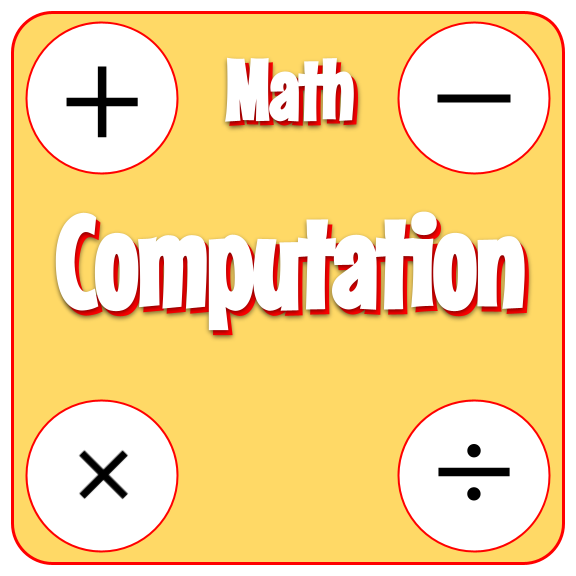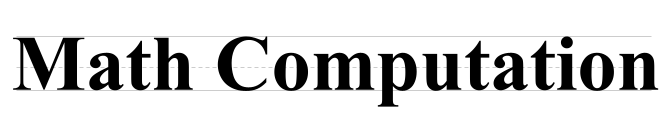Back to Mr. Anker TestsUse these practice activities to help you add, subtract, multiply, and divide like a human calculator!
Teachers & Parents: It is recommended that students complete and master each activity before moving on to the next.

 Multiplication 1Multiply one-digit factors. Multiplication 2Multiply one-digit factors. Multiplication 3Multiply one-digit factors. Multiplication 4Multiply one-digit factors.
 Multiplication 5Multiply one-digit factors. Multiplication 2'sMultiply by 2. Multiplication 3'sMultiply by 3. Multiplication 4'sMultiply by 4.
 Multiplication 5'sMultiply by 5. Multiplication 6'sMultiply by 6. Multiplication 7'sMultiply by 7. Multiplication 8'sMultiply by 8.
 Multiplication 9'sMultiply by 9. Multiplication 10'sMultiply by 10. Multiplication 11'sMultiply by 11. Multiplication 12'sMultiply by 12.
 Missing Factors 1Determine the missing factor in a multiplication equation. Missing Factors 2Determine the missing factor in a multiplication equation. Missing Factors 3Determine the missing factor in a multiplication equation. Missing Factors 4Determine the missing factor in a multiplication equation.
 Multiplication DoublesMultiply when factors up to 12 are the same. Multiplication 3 FactorsSet 1 Multiplication 3 FactorsSet 2 Multiplication with Decimal FactorsMultiply a whole number by a decimal factor.
 Mental Multiplying 1Multiply one digit by two digit factors. Mental Multiplying 2Multiply one digit by two digit factors. Mental Multiplying 3Multiply one digit by two digit factors.
 Multiplying with Zeros 1Multiply by ten. Multiplying with Zeros 2Multiply by ten. Multiplying with Zeros 3Multiply by one hundred. Multiplying with Zeros 4Multiply by one thousand.
 Multiplying with Zeros 5Multiply by various values with zeros. Multiplying with Zeros 6Multiply by various values with zeros.